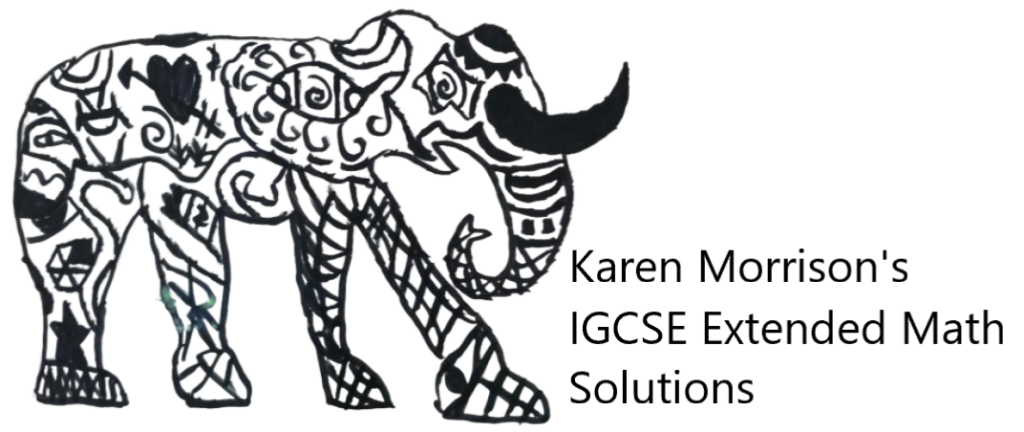# 2.5 Problems solved by linear equations

Chapter 2: Algebra 1
2.5 Problems solved by linear equations